# NCERT Solutions for Class 7 Maths Chapter 2 Fractions and Decimals Ex 2.7

NCERT Solutions for Class 7 Maths Chapter 2 Fractions and Decimals Ex 2.7

### NCERT Solutions for Class 7 Maths Chapter 2 Fractions and Decimals Ex 2.7

NCERT Solutions for Class 7 Maths Chapter 2 Fractions and Decimals Exercise 2.7
Ex 2.7 Class 7 Maths Question 1.
Find:
(i) 0.4 ÷ 2
(ii) 0.35 ÷ 5
(iii) 2.48 ÷ 4
(iv) 65.4 ÷ 6
(v) 651.2 ÷ 4
(vi) 14.49 ÷ 7
(vii) 3.96 ÷ 4
(viii) 0.80 ÷ 5
Solution: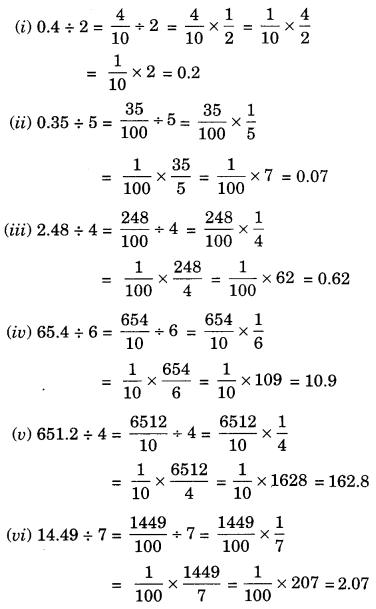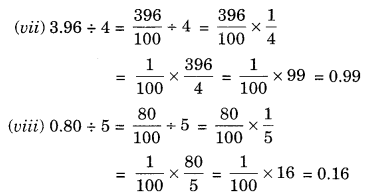Ex 2.7 Class 7 Maths Question 2.
Find:
(i) 4.8 ÷ 10
(ii) 52.5 ÷ 10
(iii) 0.7 ÷ 10
(iv) 33.1 ÷ 10
(v) 272.23 ÷ 10
(vi) 0.56 ÷ 10
(vii) 3.97 ÷10
Solution:
(i) 4.8 ÷ 10 = 0.48 (Shifting the decimal point to the left by 1 place)
(ii) 52.5 ÷ 10 = 5.25 (Shifting the decimal point to the left by 1 place)
(iii) 0.7 ÷ 10 = 0.07 (Shifting the decimal point to the left by 1 place)
(iv) 33.1 ÷ 10 = 3.31 (Shifting the decimal point to the left by 1 place)
(v) 272.23 ÷ 10 = 27.223 (Shifting the decimal point to the left by 1 place)
(vi) 0.56 4- 10 = 0.056(Shifting the decimal point to the left by 1 place)
(vii) 3.97 ÷ 10 = 0.397(Shifting the decimal point to the left by 1 place)

Ex 2.7 Class 7 Maths Question 3.
Find:
(i) 2.7 ÷ 100
(ii) 0.3 ÷ 100
(iii) 0.78 ÷ 100
(iv) 432.6 ÷ 100
(v) 23.6 ÷ 100
(vi) 98.53 ÷ 100
Solution:
Solution:
(i) 2.7 ÷ 100 = 0.027(Shifting the decimal point to the left by 2 places)
(ii) 0.3 ÷ 100 = 0.003(Shifting the decimal point to the left by 2 places)
(iii) 0.78 ÷ 100 = 0.0078 (Shifting the decimal point to the left by 2 places)
(iv) 432.6 ÷ 100 = 4.326 (Shifting the decimal point to the left by 2 places)
(v) 23.6 ÷ 100 = 0.236 (Shifting the decimal point to the left by 2 places)
(vi) 98.53 ÷ 100 = 0.9853 (Shifting the decimal point to the left by 2 places)

Ex 2.7 Class 7 Maths Question 4.
Find:
(i) 7.9 ÷ 1000
(ii) 26.3 ÷ 1000
(iii) 38.53 ÷ 1000
(iv) 128.9 ÷ 1000
(v) 0.5 ÷ 1000
Solution:
(i) 7.9 ÷ 1000 = 0.0079 (Shifting the decimal point to the left by 3 places)
(ii) 26.3 ÷ 1000 = 0.0263 (Shifting the decimal point to the left by 3 places)
(iii) 38.53 ÷ 1000 = 0.03853 (Shifting the decimal point to the left by 3 places)
(iv) 128.9 ÷ 1000 = 0.1289 (Shifting the decimal point to the left by 3 places)
(v) 0.5 ÷ 1000 = 0.0005 (Shifting the decimal point to the left by 3 places)

Ex 2.7 Class 7 Maths Question 5.
Find:
(i) 7 ÷ 3.5
(ii) 36 ÷ 0 .2
(iii) 3.25 ÷ 0.5
(iv) 30.94 ÷ 0.7
(v) 0.5 ÷ 0.25
(vi) 7.75 ÷ 0.25
(vii) 76.5 ÷ 0.15
(viii) 37.8 ÷ 1.4
(ix) 2.73 ÷ 1.3
Solution: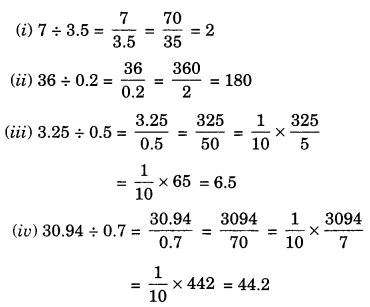Ex 2.7 Class 7 Maths Question 6.
A vehicle covers a distance of 43.2 km in 2.4 litres of Petrol. How much distance will it cover in one litre of petrol?
Solution:
2.4 litres of petrol is required to cover 43.2 km distance
∴ 1 litre of petrol will be required to cover 43.2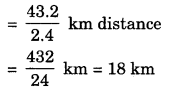Hence, the required distance = 18 km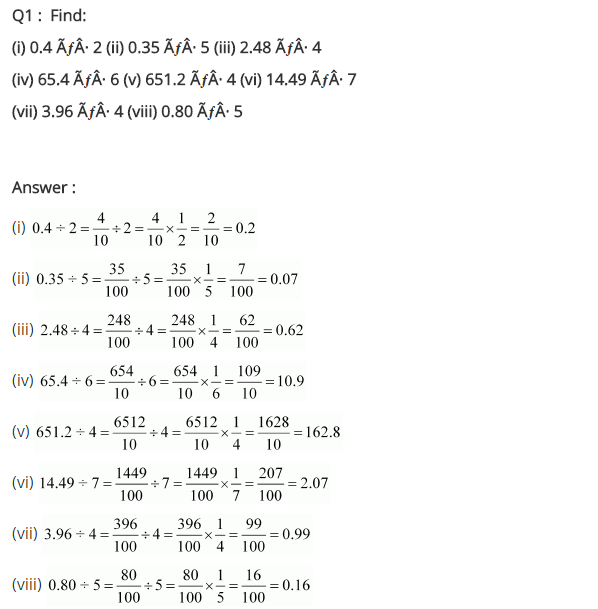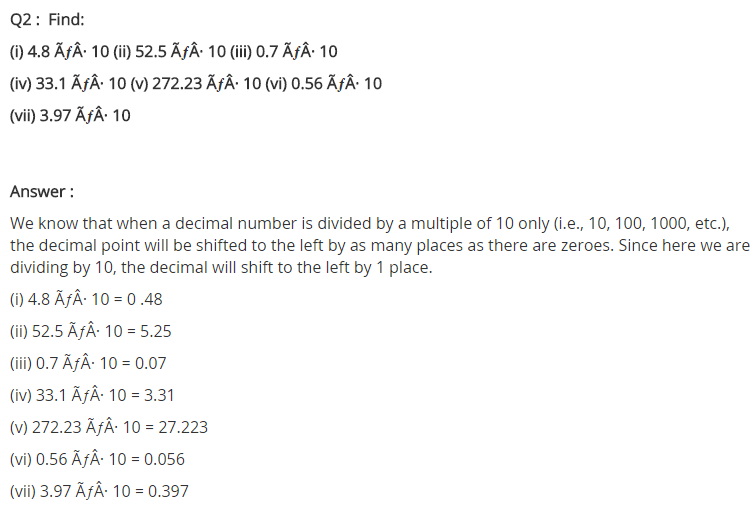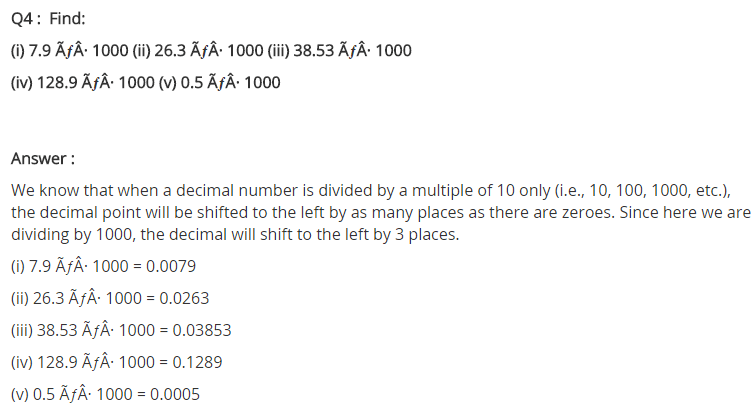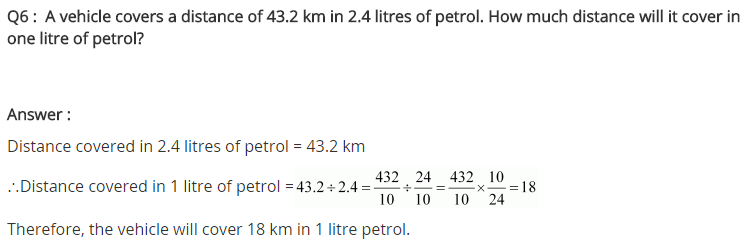## SabDekho

The Complete Educational Website